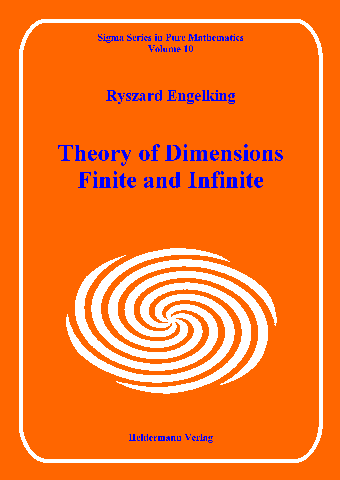SSPM
Vol 10

Catalogue of
Book Series

List of Books
in this Series

Previous Book
in this Series

Next Book
in this Series

Sigma Series in Pure Mathematics -- Volume 10Enlarged Picture

Ryszard Engelking

Theory of Dimensions: Finite and Infinite

410 pages, hard cover, ISBN 3-88538-010-2, EUR 75.00, 1995

The classical results in dimension theory, contained in the first four chapters, are followed by three chapters devoted to infinite-dimensional spaces. This is a rather new field, which has evolved however during the last 20 years into a most interesting theory presented here for the first time in concise and unified form.

The book is composed in the meanwhile famous style of the author: sections are followed by an abundance of exercises from simple to 'impossible', rounding the section in substance and leading to current topics of research.

This book is intended for students as well as for the working mathematician. It is indispensable in every mathematical library and completes the encyclopedical work of the author in topology.

"Anybody who works through this wonderful dimension theory book will not only learn some dimension theory (and want to go more deeply into the subject), but also will learn a storehouse full of the basic tools used in all of topology." (L. R. Rubin, Zentralblatt f. Mathematik).

Zentralblatt-Review

Contents:

 Preface vii Chapter 1: Dimension theory of separable metric spaces 1.1 Definition of the small inductive dimension 2 1.2 The separation and enlargement theorems for dimension 0 8 1.3 The sum, Cartesian product, universal space, compactification and embedding theorems for dimension 0 15 1.4 Various kinds of disconnectedness 24 1.5 The sum, decomposition, addition, enlargement, separation and Cartesian product theorems 31 1.6 Definitions of the large inductive dimension and the covering dimension. Metric dimension 40 1.7 The compactification and coincidence theorems. Characterization of dimensions in terms of partitions 47 1.8 Dimensional properties of Euclidean spaces and the Hilbert cube. Infinite-dimensional spaces 56 1.9 Characterization of dimension in terms of mappings to spheres. Cantor-manifolds. Cohomological dimension 69 1.10 Characterization of dimension in terms of mappings to polyhedra 79 1.11 The embedding and universal space theorems 94 1.12 Dimension and mappings 106 1.13 Dimension and inverse sequence of polyhedra 114 1.14 Axioms for dimension 123 Chapter 2: The large inductive dimension 2.1 Hereditarily normal and strongly hereditarily normal spaces 127 2.2 Basic properties of the dimension Ind in normal and hereditarily normal spaces 133 2.3 Basic properties of the dimension Ind in strongly hereditarily normal spaces 144 2.4 Relations between the dimensions ind and Ind. Cartesian product theorems for the dimension Ind. Dimension Ind and mappings 155 Chapter 3: The covering dimension 3.1 Basic properties of the dimension dim in normal spaces. Relations between the dimensions ind, Ind and dim 168 3.2 Characterizations of the dimension dim in normal spaces 182 3.3 Dimension dim and mappings 193 3.4 The compactification, universal space and Cartesian product theorems for the dimension dim. Dimension dim and inverse systems of compact spaces 205 Chapter 4: Dimension theory of metrizable spaces 4.1 Basic properties of dimension in metrizable spaces 217 4.2 Characterizations of dimension in metrizable spaces. The universal space theorems 228 4.3 Dimension and mappings in metrizable spaces 240 Chapter 5: Countable-dimensional spaces 5.1 Definitions and characterizations of countable-dimensional and strongly countable-dimensional spaces 253 5.2 Basic properties of countable-dimensional and strongly countable-dimensional spaces 261 5.3 The compactification and universal space theorems for countable-dimensional and strongly countable-dimensional spaces 271 5.4 Countable dimensionality and mappings 280 5.5 Locally finite-dimensional spaces 288 Chapter 6: Weakly infinite-dimensional spaces 6.1 Definition and basic properties of weakly infinite-dimensional spaces 300 6.2 An example of a totally disconnected strongly infinite-dimensional space 312 6.3 Weak infinite dimensionality and mappings 316 Chapter 7: Transfinite dimensions 7.1 Definitions and basic properties of the transfinite dimensions trind and trInd 325 7.2 The separation, sum, enlargement, completion and universal space theorems for the transfinite dimensions trind and trInd 338 7.3 Transfinite dimensions trker and trdim 351 Bibliography 365 List of special symbols 393 Author index 395 Subject index 398